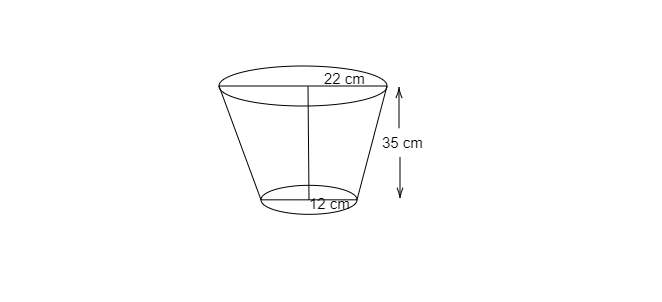# The diameters of the two circular ends of the bucket are $44\ cm$ and $24\ cm$. The height of the bucket is $35\ cm$. Find the capacity of the bucket.

Given: The diameters of the two circular ends of the bucket are $44\ cm$ and $24\ cm$. The height of the bucket is $35\ cm$.

To do: To find the capacity of the bucket.

Solution:

As given, Diameter of the upper end$=44\ cm$

Radius of the upper end, $R=\frac{44}{2}=22\ cm$

Diameter of the lower end of bucket, $24\ cm$

Radius of the lower end$r=\frac{24}{2}=12\ cm$

Height of the bucket, $h=35\ cm$Volume of frustum of cone$=\frac{\pi h}{3}( R^2+Rr+r^2)$

$1\ litre=1000\ cm^3$

Volume of a frustum cone $=\frac{\pi (35)}{3}( 22^2+12^2+( 22)( 12))$
$=32706.67\ cm^3$

$=32.7\ litres$

Thus, the capacity of the bucket is $32.7\ litres$.